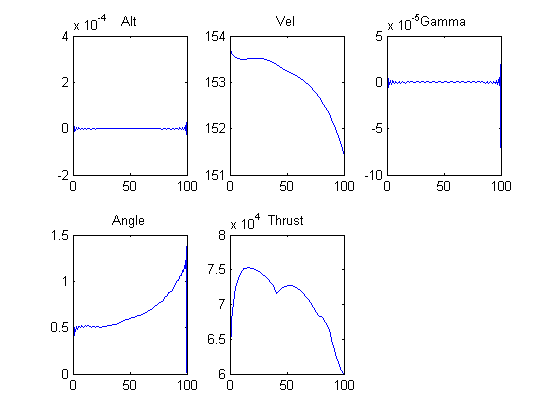36  Flight Path Tracking

Minimum Cost Optimal Control: An Application to Flight Level Tracking, John Lygeros, Department of Engineering, University of Cambridge, Cambridge, UK.

36.1  Problem Description

Find scalar w over t in [0; t_F ] to minimize:

J =
 100 0
(x32dt

subject to:

Equations in the code.

Reference: 

36.2  Problem setup

toms t
p = tomPhase('p', t, 0, 100, 60);
setPhase(p);

tomStates x1s x2 x3s
tomControls u1s u2

x1 = x1s*100;
x3 = x3s*10;
u1 = u1s*10e3;
cr2d = pi/180;

% Box constraints
cbox = {
92       <= icollocate(x1) <= 170
-20*cr2d <= icollocate(x2) <= 25*cr2d
-150     <= icollocate(x3) <= 150
60e3     <= collocate(u1) <= 120e3
-150     <= collocate(u2) <= 150};

% Boundary constraints
cbnd = initial({x1 == 153.73
x2 == 0; x3 == 0});

L = 65.3;
D = 3.18;
m = 160e3;
g = 9.81;
c = 6;

% ODEs and path constraints
ceq = collocate({
dot(x1) == (-D/m*x1.^2-g*sin(x2)+u1/m)
dot(x2) == L/m*x1.*(1-c*x2)-g*cos(x2)./x1+L*c/m*u2
dot(x3) == (x1.*sin(x2))});

% Objective
objective = integrate(x3.^2);

36.3  Solve the problem

options = struct;
options.name = 'Flight Path Tracking';
solution = ezsolve(objective, {cbox, cbnd, ceq}, [], options);
t  = subs(collocate(t),solution);
x1 = subs(collocate(x1),solution);
x2 = subs(collocate(x2),solution);
x3 = subs(collocate(x3),solution);
u1 = subs(collocate(u1),solution);
u2 = subs(collocate(u2),solution);
Problem type appears to be: qpcon
Starting numeric solver
===== * * * =================================================================== * * *
TOMLAB - Tomlab Optimization Inc. Development license  999001. Valid to 2011-02-05
=====================================================================================
Problem: ---  1: Flight Path Tracking           f_k       0.000000009789752895
sum(|constr|)      0.000000000014497456
f(x_k) + sum(|constr|)      0.000000009804250351
f(x_0)      0.000000000000000000

Solver: snopt.  EXIT=0.  INFORM=1.
SNOPT 7.2-5 NLP code
Optimality conditions satisfied

FuncEv    1 ConstrEv  444 ConJacEv  444 Iter  421 MinorIter  720
CPU time: 13.125000 sec. Elapsed time: 13.344000 sec.

36.4  Plot result

figure(1);
subplot(2,3,1);
plot(t,x3,'-');
title('Alt')
subplot(2,3,2);
plot(t,x1,'-');
title('Vel')
subplot(2,3,3);
plot(t,x2,'-');
title('Gamma')
subplot(2,3,4);
plot(t,u2,'-');
title('Angle')
subplot(2,3,5);
plot(t,u1,'-');
title('Thrust')